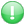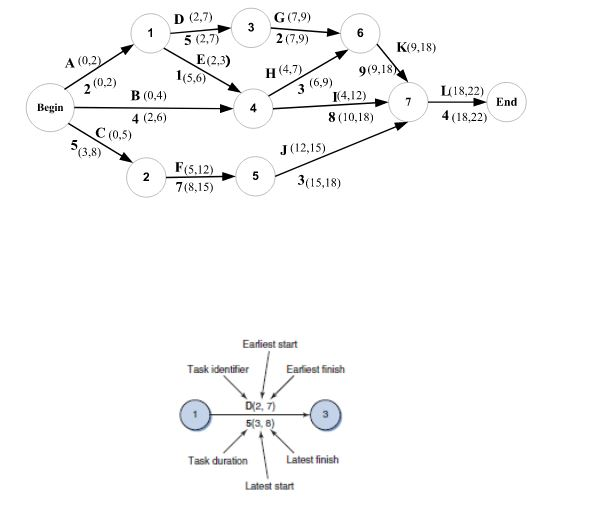Get premium membership and access questions with answers, video lessons as well as revision papers.

# Define the methods used to find the critical path

Define the methods used to find the critical path1. Calculate slack for each activity on the WBS using a network diagram, or
2. Find the longest path through the network diagram
Example: Find the Critical Patha) Find Critical Path by Calculating Slack
- The calculation is performed for each activity in two passes through the entire network diagram for each path.
- The first pass through starts at the begin node and moves to the end node through each path calculating the ES (Earliest Start time) and EF (Earliest Finish time).
- The second pass through starts at the end node and moves to the beginning node through each path calculating LS (Latest Finish time) and LF (Latest Finish Time)
- Those activities with zero slack are on the critical path

b) Find the Longest Path
Path 1 = A, D, G, K, L = 22 days
Path 2 = A, E, H, K, L = 19
Path 3 = A, E, I, L = 15
Path 4 = B, H, K, L = 20
Path 5 = B, I, L = 16
Path 6 = C, F, J, L = 19
Path A, D, G, K, L is the critical path duration is longest

Critical Path
- If one of more activities on the critical path takes longer than planned, the whole project schedule will slip unless
corrective action is taken

Misconceptions:
- The critical path is not the one with all the critical activities; it only accounts for time
- The critical path can change as the project progresses!!!!!!!!!
francis1897 answered the question on March 14, 2023 at 07:28